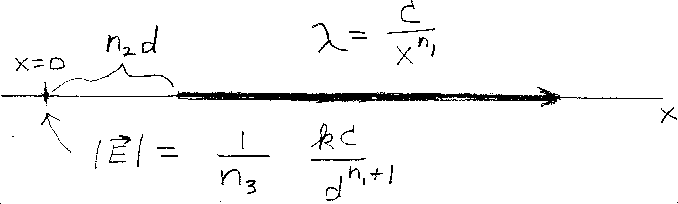Problem A12: Consider the long thin semi-infinite non-conducting rod shown in the figure above. It lies on the x-axis with the left end located a distance n2d from the origin. The linear charge density of the rod decreases as 1/xn1 where x is the distance from the origin. The expression for the linear charge density is &lambda = C/xn1, where C is a constant. What is the magnitude of the electric field at the origin? That is if |E| = (1/n3) kC/dn1+1, what is n3?n1 = n2 = Input n3:

If you are currently in my class, you can record your grade by entering your name and student ID number (without the leading zeros) below and clicking on "record grade".
 First Name = Last Name = ID = Problem: